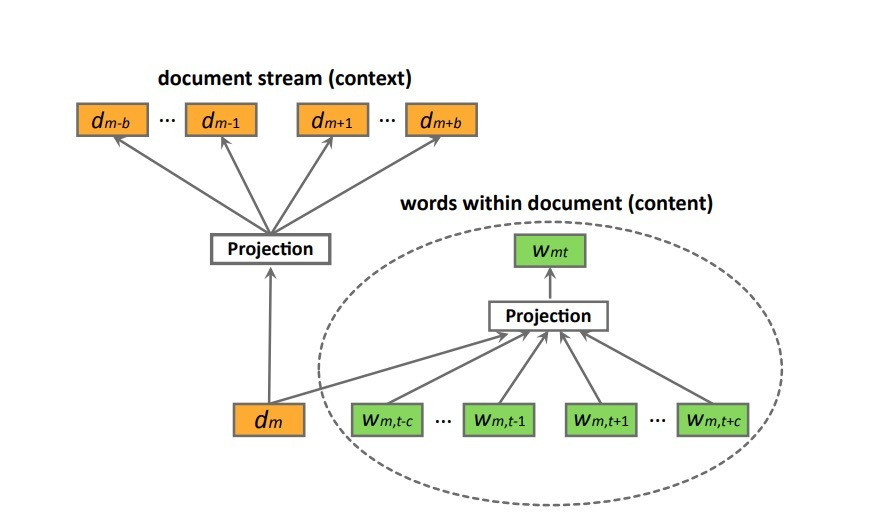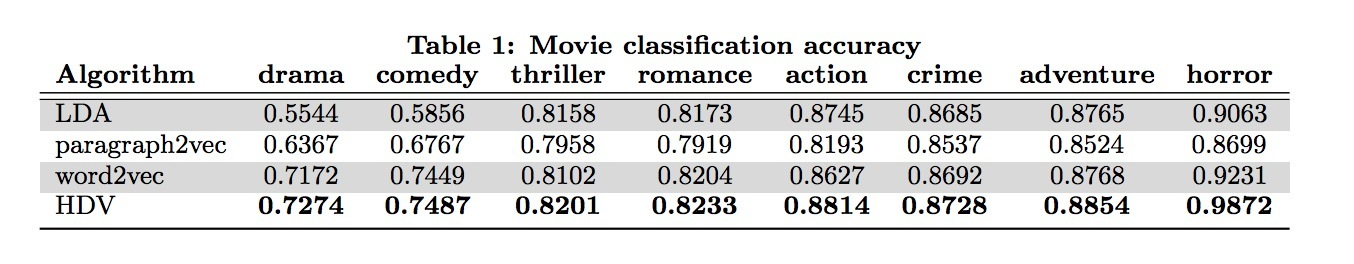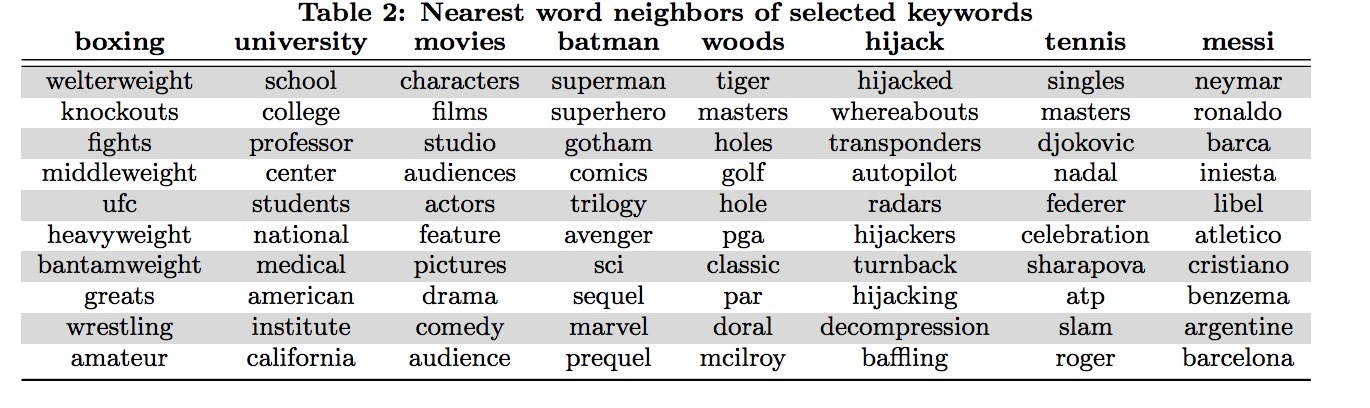# 3.层次化语言模型

## 3.1 模型结构$L = \sum_{s \in S} (\alpha \sum_{d_m \in s} \sum_{w_{mt} \in d_m} log P(w_{mt} | w_{m,t-c}: w_{m,t+c}, d) + \\ \sum _{d_m \in s} (\alpha log P(d_m | w_{m1}: w_{mT}) + \sum_{-b \le i \le b, i \neq 0} log P(d_{m+i} | d_m)))$ …(8)

$P(d_{m+i} | d_{m}) = \frac{exp(v_{d_m}^T v_{d_{m+i}}')} {\sum_{d=1}^{M} exp(v_{d_m}^T v_d')}$ …(9)

$P(w_{mt} | w_{m,t-c}: w_{m,t+c}, d_m) = \frac{exp(\bar{v}^T v_{w_{mt}}')} {\sum_{w=1}^{W} exp(\bar{v}^T v_w')}$ …(10)

$\bar{v} = \frac{1}{2c+1} (v_{d_m} + \sum_{-c \leq j \leq c, j \neq 0} v_{w_{m,t+j}})$ …(11)

## 3.3 模型最优化

$P(d_{m+i} \mid d_m) = \prod_l P(h_l \mid q_l, d_m)$ …(12)

$P(h_l = 1 \mid q_l, d_m) = \sigma(v_{d_m}^T V_{q_l})$ …(13)Updated on